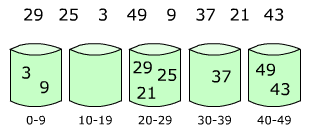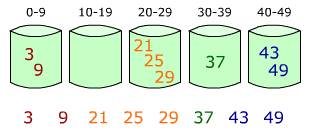# Bucket sort parallel algorithm using C++ OpenMPI

Bucket Sort is quite an easy to implement algorithm when talking about parallel algorithms. Its main logic is to spare different parts of array into different buckets, then sort them at the same time with another algorithm and put it back together in one big result array.

I took some images from Wikipedia for better understanding:We put those numbers into buckets based on interval which is get looping from the min value until the end of all numbers which is the max number in the array. In this case you might see that all the negative numbers will be in the first bucket which might be in some case a disadvantage of this particular implementation of this algorithm.

The numbers are at first calculated by the same logic and the main process sends information of how many numbers each bucket will need to parse and get. By doing the same routine again, the main process sends out all the numbers to a particular bucket and buckets are receiving information until it gets all the numbers that should be sent to it.After putting the numbers into the specific buckets, you just sort them out using any sorting algorithm you want. The native sort() function of C++ is used in this example.

I’ve even create a simple “random” numbers generator which you can use to create some test info. The code is below:

```
#include
#include
#include

using namespace std;

int main(int argc, char* argv[])
{
int size;
cout << "Kokio dydzio masyva sugeneruot?" << endl; 	cin >> size;
unsigned int *array = new unsigned int [size];
unsigned int c;
srand(time(nullptr));	// preparing random function
//srand(1); // for the same results

for(c = 0 ; c < size ; c++)	//
array = rand();
ofstream fd("testing_info.txt");
fd << size << endl;
for(c = 0 ; c < size ; c++)	{
fd << array << endl;
}
fd.close();
return 0;
}

```

At the main code of the Bucket sort algorithm implementation using C++ Open MPI:

```
#include "mpi.h"
#include <iostream>
#include <fstream>
#include <string>
#include <iomanip>
#include <cstdlib>
#include <ctime>
#include <algorithm>
#include <assert.h>

using namespace std;

int main( int argc, char* argv[] )
{
double starttime, endtime;
int proceso_id;
char processor_name[MPI_MAX_PROCESSOR_NAME];
int namelen;
int numprocsused;
// Intiating parallel part
MPI_Status stat;
MPI::Init();
/
MPI_Comm_size(MPI_COMM_WORLD, &numprocsused);
proceso_id = MPI::COMM_WORLD.Get_rank();
MPI_Get_processor_name(processor_name, &namelen);
if(proceso_id == 0) {
// if it is main process

// --- We are getting info from text file
int SIZE;
unsigned int value;
ifstream fd("testing_ingo.txt");
fd >> SIZE; // the first number on the first line is the number of numbers in file
unsigned int *array = new unsigned int [SIZE];
for(int c = 0 ; c < SIZE ; c++)	{
fd >> value;
array = value;
}
// --- DEBUG: in order to check if information was read properly
//for(int c = 0; c < SIZE; c++) {
//	printf("   %d   ", array);
//	if((c+1)%5 == 0 )
//		printf("\n",SIZE);
//}

// starting time calculation of the sort
starttime = MPI_Wtime();

// min and max values are got
unsigned int min = array;
unsigned int max = array;
for(int i=0; i < SIZE; i++) {
if(array[i] < min) { min = array[i]; }
if(array[i] > max) { max = array[i]; }
}

// calculating how many numbers each bucket/process will get numbers
int *elementQtyArray = new int[numprocsused]; /
// default values
for(int d=1; d < numprocsused; d++) {
elementQtyArray[d] = 0;
}
for(int d=0; d < SIZE; d++) {
int increaseOf = max/(numprocsused-1);
int iteration = 1;
bool pridetas = false;
for(unsigned int j = increaseOf; j <= max; j = j + increaseOf) {
if(array[d] <= j) {
elementQtyArray[iteration]++;
pridetas = true;
break;
}
iteration++;
}
if (!pridetas) {
elementQtyArray[iteration-1]++;
}
}

// Sending how many each process/bucket will get numbers
for(int i=1; i<numprocsused; i++) {
MPI_Send(&elementQtyArray[i], 1, MPI_INT, i, -2, MPI_COMM_WORLD);
}

// doing the same, this time sending the numbers
for(int d=0; d < SIZE; d++) {
int increaseOf = max/(numprocsused-1);
int iteration = 1;
bool issiunte = false;
for (unsigned int j = increaseOf; j <= max; j = j + increaseOf) {
if(array[d] <= j) {
MPI_Send(&array[d], 1, MPI_UNSIGNED, iteration, -4, MPI_COMM_WORLD);
issiunte = true;
break;
}
iteration++;
}
if (!issiunte) {
MPI_Send(&array[d], 1, MPI_UNSIGNED, iteration-1, -4, MPI_COMM_WORLD);
}
}

// Getting back results and adding them to one array
int lastIndex = 0; int indexi = 0;
for(int i=1; i < numprocsused; i++) {
unsigned int * recvArray = new unsigned int [elementQtyArray[i]];
MPI_Recv(&recvArray, elementQtyArray[i], MPI_UNSIGNED, i, 1000, MPI_COMM_WORLD, &stat);
if(lastIndex == 0) {
lastIndex = elementQtyArray[i];
}
for(int j=0; j<elementQtyArray[i]; j++) {
array[indexi] = recvArray[j];
indexi++;
}
}

// stoping the time
endtime   = MPI_Wtime();

// showing results in file
ofstream fr("results.txt");
for(int c = 0 ; c < SIZE ; c++)	{
fr << array << endl;
}
fr.close();

// sorting results
printf("it took %f seconds \n", endtime-starttime);
printf("Numbers: %d \n", SIZE);
printf("Processes:  %d \n", numprocsused);
//----------------------------------------------------------------------------------------------------------------
} else {
// if child process
int elementQtyUsed; // kiek elementu si gija gauja is tevinio proceso
// --- getting the number of numbers in the bucket
MPI_Recv(&elementQtyUsed, 1, MPI_INT, 0, -2, MPI_COMM_WORLD, &stat);

unsigned int *localArray = new unsigned int [elementQtyUsed]; // initiating a local bucket

// --- getting numbers from the main process
for(int li = 0; li < elementQtyUsed; li++) {
MPI_Recv(&receivedElement, 1, MPI_UNSIGNED, 0, -4, MPI_COMM_WORLD, &stat);
}

// --- sorting the bucket
sort(localArray, localArray+elementQtyUsed);

// --- sending back sorted array
MPI_Send(localArray, elementQtyUsed, MPI_UNSIGNED, 0, 1000, MPI_COMM_WORLD);
}

MPI::Finalize();

return 0;
}

```

Do not forget that you need to have OpenMPI configured and installed in your machine in order to have this code work. There’re some tutorials on the Internet how you can add it to Visual Studio.